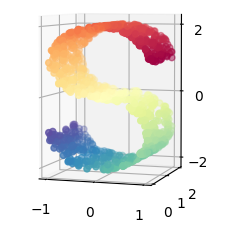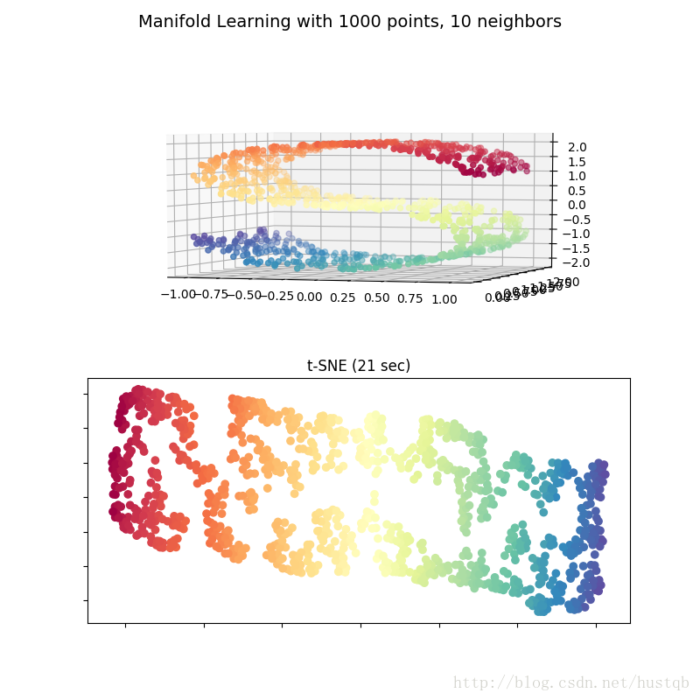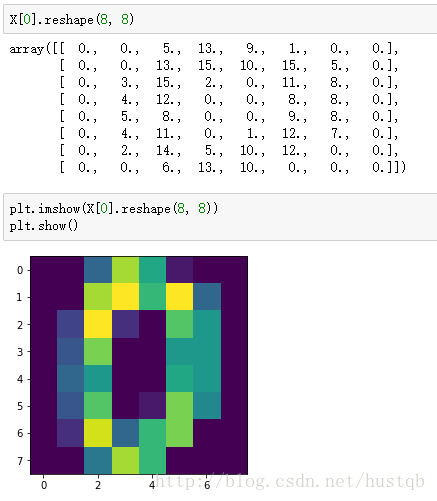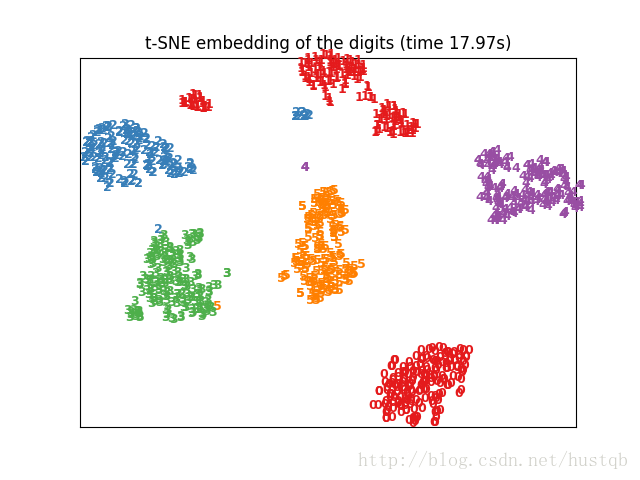# 数据降维与可视化之t-SNE

t-SNE是目前来说效果最好的数据降维与可视化方法，但是它的缺点也很明显，比如：占内存大，运行时间长。但是，当我们想要对高维数据进行分类，又不清楚这个数据集有没有很好的可分性（即同类之间间隔小，异类之间间隔大），可以通过t-SNE投影到2维或者3维的空间中观察一下。如果在低维空间中具有可分性，则数据是可分的；如果在高维空间中不具有可分性，可能是数据不可分，也可能仅仅是因为不能投影到低维空间。

t-distributed Stochastic Neighbor Embedding(t-SNE)

t-SNE（TSNE）将数据点之间的相似度转换为概率。原始空间中的相似度由高斯联合概率表示，嵌入空间的相似度由“学生t分布”表示。• t-SNE的计算复杂度很高，在数百万个样本数据集中可能需要几个小时，而PCA可以在几秒钟或几分钟内完成
• Barnes-Hut t-SNE方法（下面讲）限于二维或三维嵌入。
• 算法是随机的，具有不同种子的多次实验可以产生不同的结果。虽然选择loss最小的结果就行，但可能需要多次实验以选择超参数。
• 全局结构未明确保留。这个问题可以通过PCA初始化点（使用`init ='pca'`）来缓解。

### 优化 t-SNE

t-SNE的主要目的是高维数据的可视化。因此，当数据嵌入二维或三维时，效果最好。有时候优化KL散度可能有点棘手。有五个参数可以控制t-SNE的优化，即会影响最后的可视化质量：

• perplexity困惑度
• early exaggeration factor前期放大系数
• learning rate学习率
• maximum number of iterations最大迭代次数
• angle角度

### Barnes-Hut t-SNE

Barnes-Hut t-SNE主要是对传统t-SNE在速度上做了优化，是现在最流行的t-SNE方法，同时它与传统t-SNE还有一些不同：

• Barnes-Hut仅在目标维度为3或更小时才起作用。以2D可视化为主。
• Barnes-Hut仅适用于密集的输入数据。稀疏数据矩阵只能用特定的方法嵌入，或者可以通过投影近似，例如使用sklearn.decomposition.TruncatedSVD
• Barnes-Hut是一个近似值。使用angle参数对近似进行控制，因此当参数`method="exact"`时，`TSNE()`使用传统方法，此时angle参数不能使用。
• Barnes-Hut可以处理更多的数据。 Barnes-Hut可用于嵌入数十万个数据点。

### 注意事项

• 数据集在所有特征维度上的尺度应该相同

### 参数说明

parametersdescription
n_componentsint, 默认为2，嵌入空间的维度（嵌入空间的意思就是结果空间）
perplexityfloat, 默认为30，数据集越大，需要参数值越大，建议值位5-50
early_exaggerationfloat, 默认为12.0，控制原始空间中的自然集群在嵌入式空间中的紧密程度以及它们之间的空间。 对于较大的值，嵌入式空间中自然群集之间的空间将更大。 再次，这个参数的选择不是很关键。 如果在初始优化期间成本函数增加，则可能是该参数值过高。
learning_ratefloat, default:200.0, 学习率，建议取值为10.0-1000.0
n_iterint, default:1000, 最大迭代次数
n_iter_without_progressint, default:300, 另一种形式的最大迭代次数，必须是50的倍数
metricstring or callable, 精确度的计量方式
initstring or numpy array, default:”random”, 可以是’random’, ‘pca’或者一个numpy数组(shape=(n_samples, n_components)。
verboseint, default:0, 训练过程是否可视
random_stateint, RandomState instance or None, default:None，控制随机数的生成
methodstring, default:’barnes_hut’, 对于大数据集用默认值，对于小数据集用’exact’
anglefloat, default:0.5, 只有`method='barnes_hut'`时可用

attributesdescription
embedding_嵌入向量
kl_divergence最后的KL散度
n_iter_迭代的次数

Methodsdescription
fit将X投影到一个嵌入空间
fit_transform将X投影到一个嵌入空间并返回转换结果
get_params获取t-SNE的参数
set_params设置t-SNE的参数

### 实例

#### Hello World

```import numpy as np
from sklearn.manifold import TSNE
X = np.array([[0, 0, 0], [0, 1, 1], [1, 0, 1], [1, 1, 1]])
tsne = TSNE(n_components=2)
tsne.fit_transform(X)
print(tsne.embedding_)
'''输出
[[   3.17274952 -186.43092346]
[  43.70787048 -283.6920166 ]
[ 100.43157196 -145.89025879]
[ 140.96669006 -243.15138245]]'''```

#### S曲线的降维与可视化

S曲线上的数据是高维的数据，其中不同颜色表示数据的不同类别。当我们通过t-SNE嵌入到二维空间中后，可以看到数据点之间的类别信息完美的保留了下来

```# coding='utf-8'
"""# 一个对S曲线数据集上进行各种降维的说明。"""
from time import time

import matplotlib.pyplot as plt
from mpl_toolkits.mplot3d import Axes3D
from matplotlib.ticker import NullFormatter

from sklearn import manifold, datasets

# # Next line to silence pyflakes. This import is needed.
# Axes3D

n_points = 1000
# X是一个(1000, 3)的2维数据，color是一个(1000,)的1维数据
X, color = datasets.samples_generator.make_s_curve(n_points, random_state=0)
n_neighbors = 10
n_components = 2

fig = plt.figure(figsize=(8, 8))
# 创建了一个figure，标题为"Manifold Learning with 1000 points, 10 neighbors"
plt.suptitle("Manifold Learning with %i points, %i neighbors"
% (1000, n_neighbors), fontsize=14)

'''绘制S曲线的3D图像'''
ax.scatter(X[:, 0], X[:, 1], X[:, 2], c=color, cmap=plt.cm.Spectral)
ax.view_init(4, -72)  # 初始化视角

'''t-SNE'''
t0 = time()
tsne = manifold.TSNE(n_components=n_components, init='pca', random_state=0)
Y = tsne.fit_transform(X)  # 转换后的输出
t1 = time()
print("t-SNE: %.2g sec" % (t1 - t0))  # 算法用时
plt.scatter(Y[:, 0], Y[:, 1], c=color, cmap=plt.cm.Spectral)
plt.title("t-SNE (%.2g sec)" % (t1 - t0))
ax.xaxis.set_major_formatter(NullFormatter())  # 设置标签显示格式为空
ax.yaxis.set_major_formatter(NullFormatter())
# plt.axis('tight')

plt.show()```#### 手写数字的降维可视化t-SNE将8*8即64维的数据降维成2维，并在平面图中显示，这里只选取了0-5，6个手写数字。

```# coding='utf-8'
"""t-SNE对手写数字进行可视化"""
from time import time
import numpy as np
import matplotlib.pyplot as plt

from sklearn import datasets
from sklearn.manifold import TSNE

def get_data():
data = digits.data
label = digits.target
n_samples, n_features = data.shape
return data, label, n_samples, n_features

def plot_embedding(data, label, title):
x_min, x_max = np.min(data, 0), np.max(data, 0)
data = (data - x_min) / (x_max - x_min)

fig = plt.figure()
ax = plt.subplot(111)
for i in range(data.shape):
plt.text(data[i, 0], data[i, 1], str(label[i]),
color=plt.cm.Set1(label[i] / 10.),
fontdict={'weight': 'bold', 'size': 9})
plt.xticks([])
plt.yticks([])
plt.title(title)
return fig

def main():
data, label, n_samples, n_features = get_data()
print('Computing t-SNE embedding')
tsne = TSNE(n_components=2, init='pca', random_state=0)
t0 = time()
result = tsne.fit_transform(data)
fig = plot_embedding(result, label,
't-SNE embedding of the digits (time %.2fs)'
% (time() - t0))
plt.show(fig)

if __name__ == '__main__':
main()```• 版权声明 本文源自 hustqb 整理 发表
• 转载请务必保留本文链接：https://www.plob.org/article/15971.html